# Hexbyte News Computers The One On Dynamic Programming! | Blogarithms

Hexbyte News Computers

Dynamic programming (DP, as I’ll refer to it here on) is a toughie.

I’ve heard a lot of friends and juniors complain about dynamic programming and about how non-intuitive it is.

Plus, problems on DP are pretty standard in most product-company-based hiring challenges, so it seems like a good topic to address on a blog based on algorithms.

Here’s a heads-up to you though, since this article is the first in (maybe, depending on its reception), a multi-part series on DP, this article will focus on setting the groundwork of DP and I’ll dedicate most of this article to explaining how to arrive at the solutions to classical DP problems, and the general pattern of thought that I use when it comes to DP.

This article, I’ll be tackling the following –

• Coin Change Problem
• 0-1 Knapsack Problem
• DP on grids

And I’ll be leaving some more classical variants of DP as exercises.

If you’re already fully confident of why/how the above DP algorithms work correctly, then reading this article may yield little value, and you may be better off waiting for the next article on DP. 🙂

Should I add future articles, I’ll tackle some non-classical variants of DP, leveraging intuition and logic.

Onto the article!

### DP: An Intro

I’ve found myself solving the nicest of DP questions when I leverage recursion.

This is something I’ve often noticed in general, that for me, recursion is very intuitive.

While DP at times does seem confusing, I’ve found from personal experience that thinking of solutions and problems recursively makes reaching a DP solution that much easier.

Let’s take an example –

##### The Coin Change Problem

Let’s say you have an infinite amount of `K` different denominations of coins.

You need to find the minimum number of coins to possess a value of N dollars.

For example, if `K = 2` and your denominations are `{1, 2}`, and you want to find the min. number of coins needed to generate `N=9` dollars.

Here, it’s pretty obvious that’d be `5` coins (`4` two dollar coins + `1` one dollar coin).

Note: A greedy algorithm of selecting the largest available denomination under `N` at every instant won’t give optimal answers.

Try out the test case: `K=3`, `denominations = {1, 3, 4}` and `N = 10`.

The greedy answer yields 4 coins (`4` + `4` + `1` + `1`) while the optimal answer is 3 coins (`4` + `3` + `3`).

Thus, clearly we need to check all possible coin selections we can make.

Now let’s model the problem recursively.

Let’s define a mathematical function `f` where `f(N)` tells me the minimum number of coins I need to generate a value of `N`.

Let’s say I have `K` denominations.

Now, if I were to take away a coin from my value of `N`, this coin could be of any denomination, correct?

Basically, `N` could be re-written as `((N-denom[i]) + denom[i])` for any valid `0<=i.`

`I also know, that denom[i] can be represented as 1 coin (a coin whose value equals denom[i]).`

`Thus, f(N) = f(N-denom[i]) + 1`

`This is our general case.`

`What’s our base case?`

`If N=0, we need 0 coins.`

`Similarly, if N<0, its an impossible case, which needs to be handled.`

`Compiling all this, we have:`

``````
>>> def f(n, denom):
ans = 10**10
if n<=0:
return 0
for i in denom:
if n-i>=0:
ans = min(ans, 1+f(n-i, denom))
return ans

>>> f(10, [1,3,4])
3

``````

`Yay!`

`Now there are a couple of things we need to ensure we’re handling, if we want to port this to DP.`

• `No global variables should be modifed in the function`
• `Simple, memorizable states (state-space reduction)`

`And… I’d say that’s it?`

`Now, we can make denom a global array as we’re not changing it.`

`This makes our function just have one parameter. The current value, N.`

`Thus, we have an O(N*K) DP solution to this, as follows -`

``````
>>> dp = [-1 for i in range(n)]
>>> def f(n):
if dp[n]!=-1:
return dp[n]
ans = 10**10
if n<=0:
return 0
for i in denom:
if n-i>=0:
ans = min(ans, 1+f(n-i))
dp[n] = ans
return ans

``````

`And that’s it!`

##### `The 0-1 Knapsack Problem`

`Okay, so let’s say you’re a burglar who has a bag (knapsack) that can carry a total weight of W.`

`There are N valuable objects in the house you broke into.`

`Each object i, has a weight of wt[i] and a value of vals[i].`

`Your task as a burglar is to devise an efficient algorithm that can maximize the net value of objects you can steal from the house provided you place them all in your knapsack.`

`I hope you don’t have to apply this in seriousness. 😛`

`So, let’s think recursively here.`

`In general, whenever problems involve multiple solutions where there’s an “option” to choose or ignore an element, I find it suitable to use the current index of the element as a parameter.`

`So this means that my f() method already has one parameter - int idx`

`Now let’s think about what our other parameter could be by simulating our burglary.`

`At any point, when we’re looking at the object at index idx, we have a choice - to take it, or to not take it.`

`The point of DP is to optimize our solution of “checking all possibilities”, without repeating checking already checked possibilities, right?`

`So, we have a choice – take the current object, or ignore it.`

`But let’s say we do take it – we still need to verify if our knapsack can hold it, right?`

`Lets look at this recursively.`

`To make sure our current possibility is valid, and to provide insight into our decision as to whether or not can we afford to take the next object, we NEED to know our current knapsack capacity that’s left.`

`So, our states are (current_index, weight_left).`

`Let’s see how we can model this as a recursive equation now -`

``````
f(current_index, weight_left) = max( f(current_index + 1, weight_left), vals[current_index] + f(current_index+1, weight_left - wt[current_index]) )

``````

`Look closely to the right-hand-side of that equation.`

`We’re stating:`

`f(idx, weight) = max( possibility where we don’t take current element, possibility where we do take current element )`

`It’s important to note that we’ve defined f(idx, weight) to be the maximum value we can obtain assuming we’re at index idx with weight weight left over.`

`Also, pay attention to the states in the latter possibility, we even update the weight_lef`

`Read More`

``````

# `Hexbyte Hacker News Computers New for AWS Lambda – Use Any Programming Language and Share Common Components | Amazon Web Services`

`Hexbyte Hacker News Computers`

`I remember the excitement when AWS Lambda was announced in 2014! Four years on, customers are using Lambda functions for many different use cases. For example, iRobot is using AWS Lambda to provide compute services for their Roomba robotic vacuum cleaners, Fannie Mae to run Monte Carlo simulations for millions of mortgages, Bustle to serve billions of requests for their digital content.`

`Today, we are introducing two new features that are going to make serverless development even easier:`

• `Lambda Layers, a way to centrally manage code and data that is shared across multiple functions.`
• `Lambda Runtime API, a simple interface to use any programming language, or a specific language version, for developing your functions.`

`These two features can be used together: runtimes can be shared as layers so that developers can pick them up and use their favorite programming language when authoring Lambda functions.`

`Let’s see how they work more in detail.`

`Lambda Layers`

`When building serverless applications, it is quite common to have code that is shared across Lambda functions. It can be your custom code, that is used by more than one function, or a standard library, that you add to simplify the implementation of your business logic.`

`Previously, you would have to package and deploy this shared code together with all the functions using it. Now, you can put common components in a ZIP file and upload it as a Lambda Layer. Your function code doesn’t need to be changed and can reference the libraries in the layer as it would normally do.`

`Layers can be versioned to manage updates, each version is immutable. When a version is deleted or permissions to use it are revoked, functions that used it previously will continue to work, but you won’t be able to create new ones.`

`In the configuration of a function, you can reference up to five layers, one of which can optionally be a runtime. When the function is invoked, layers are installed in /opt in the order you provided. Order is important because layers are all extracted under the same path, so each layer can potentially overwrite the previous one. This approach can be used to customize the environment. For example, the first layer can be a runtime and the second layer adds specific versions of the libraries you need.`

`The overall, uncompressed size of function and layers is subject to the usual unzipped deployment package size limit.`

`Layers can be used within an AWS account, shared between accounts, or shared publicly with the broad developer community.`

`There are many advantages when using layers. For example, you can use Lambda Layers to:`

• `Enforce separation of concerns, between dependencies and your custom business logic.`
• `Make your function code smaller and more focused on what you want to build.`
• `Speed up deployments, because less code must be packaged and uploaded, and dependencies can be reused.`

`Based on our customer feedback, and to provide an example of how to use Lambda Layers, we are publishing a public layer which includes NumPy and SciPy, two popular scientific libraries for Python. This prebuilt and optimized layer can help you start very quickly with data processing and machine learning applications.`

`In addition to that, you can find layers for application monitoring, security, and management from partners such as Datadog, Epsagon, IOpipe, NodeSource, Thundra, Protego, PureSec, Twistlock, Serverless, and Stackery.`

`Using Lambda Layers`

`In the Lambda console I can now manage my own layers:`

`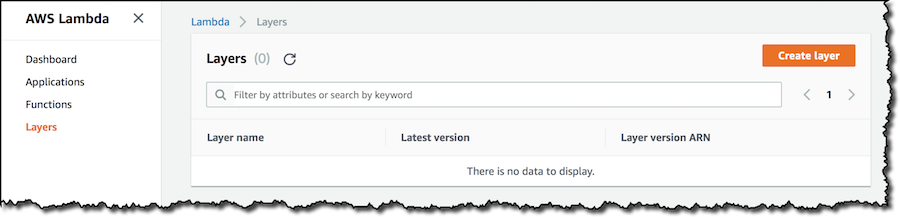`

`I don’t want to create a new layer now but use an existing one in a function. I create a new Python function and, in the function configuration, I can see that there are no referenced layers. I choose to add a layer:`

`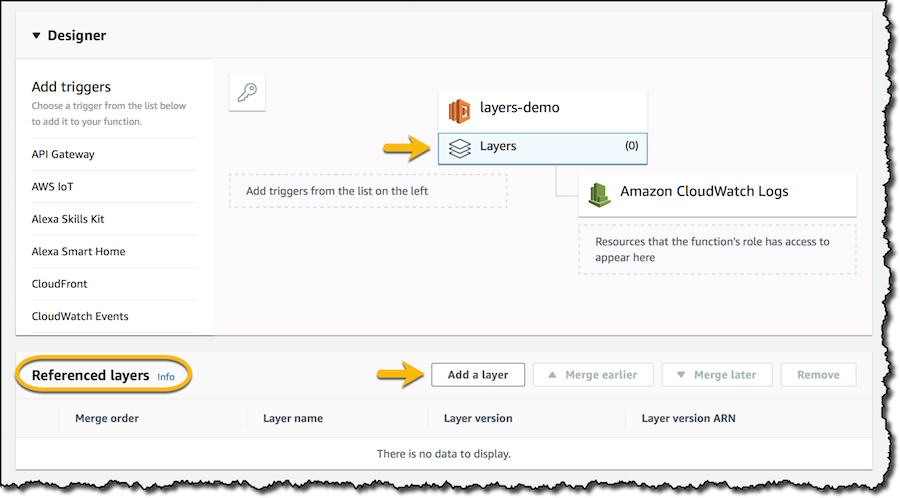`

`From the list of layers compatible with the runtime of my function, I select the one with NumPy and SciPy, using the latest available version:`

`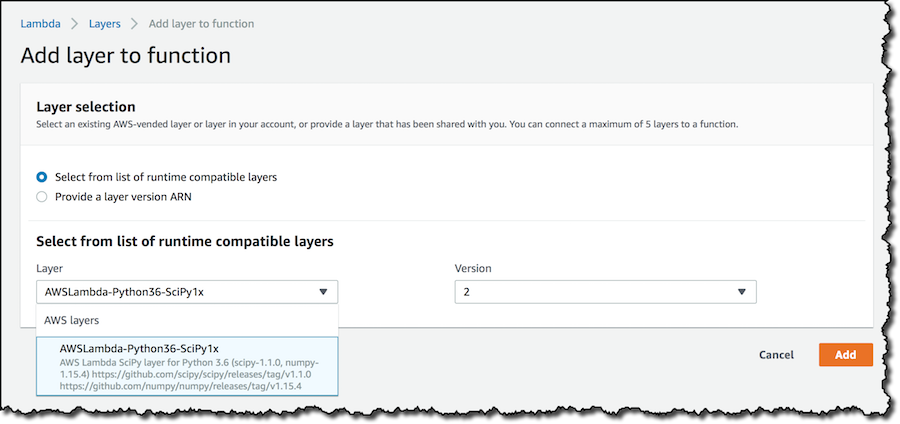`

`After I add the layer, I click Save to update the function configuration. In case you’re using more than one layer, you can adjust here the order in which they are merged with the function code.`

`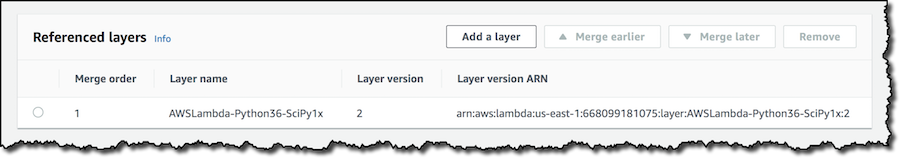`

`To use the layer in my function, I just have to import the features I need from NumPy and SciPy:`

``````import numpy as np
from scipy.spatial import ConvexHull

def lambda_handler(event, context):

print("nUsing NumPyn")

print("random matrix_a =")
matrix_a = np.random.randint(10, size=(4, 4))
print(matrix_a)

print("random matrix_b =")
matrix_b = np.random.randint(10, size=(4, 4))
print(matrix_b)

print("matrix_a * matrix_b = ")
print(matrix_a.dot(matrix_b)
print("nUsing SciPyn")

num_points = 10
print(num_points, "random points:")
points = np.random.rand(num_points, 2)
for i, point in enumerate(points):
print(i, '->', point)

hull = ConvexHull(points)
print("The smallest convex set containing all",
num_points, "points has", len(hull.simplices),
"sides,nconnecting points:")
for simplex in hull.simplices:
print(simplex, '<->', simplex)``````

`I run the function, and looking at the logs, I can see some interesting results.`

`First, I am using NumPy to perform matrix multiplication (matrices and vectors are often used to represent the inputs, outputs, and weights of neural networks):`

``````random matrix_1 =
[[8 4 3 8]
[1 7 3 0]
[2 5 9 3]
[6 6 8 9]]
random matrix_2 =
[[2 4 7 7]
[7 0 0 6]
[5 0 1 0]
[4 9 8 6]]
matrix_1 * matrix_2 =
[[ 91 104 123 128]
[ 66 4 10 49]
[ 96 35 47 62]
[130 105 122 132]]``````

`Then, I use SciPy advanced spatial algorithms to compute something quite hard to build by myself: finding the smallest “convex set” containing a list of points on a plane. For example, this can be used in a Lambda function receiving events from multiple geographic locations (corresponding to buildings, customer locations, or devices) to visually “group” similar events together in an efficient way:`

``````10 random points:
0 -> [0.07854072 0.91912467]
1 -> [0.11845307 0.20851106]
2 -> [0.3774705 0.62954561]
3 -> [0.09845837 0.74598477]
4 -> [0.32892855 0.4151341 ]
5 -> [0.00170082 0.44584693]
6 -> [0.34196204 0.3541194 ]
7 -> [0.84802508 0.98776034]
8 -> [0.7234202 0.81249389]
9 -> [0.52648981 0.8835746 ]
The smallest convex set containing all 10 points has 6 sides,
connecting points:
1 <-> 5
0 <-> 5
0 <-> 7
6 <-> 1
8 <-> 7
8 <-> 6``````

`When I was building this example, there was no need to install or package dependencies. I could quickly iterate on the code of the function. Deployments were very fast because I didn’t have to include large libraries or modules.`

`To visualize the output of SciPy, it was easy for me to create an additional layer to import matplotlib, a plotting library. Adding a few lines of code at the end of the previous function, I can now upload to Amazon Simple Storage Service (S3) an image that shows how the “convex set” is wrapping all the points:`

``````    plt.plot(points[:,0], points[:,1], 'o')
for simplex in hull.simplices:
plt.plot(points[simplex, 0], points[simplex, 1], 'k-')

img_data = io.BytesIO()
plt.savefig(img_data, format='png')
img_data.seek(0)

s3 = boto3.resource('s3')
bucket = s3.Bucket(S3_BUCKET_NAME)
bucket.put_object(Body=img_data, ContentType='image/png', Key=S3_KEY)

plt.close()``````

`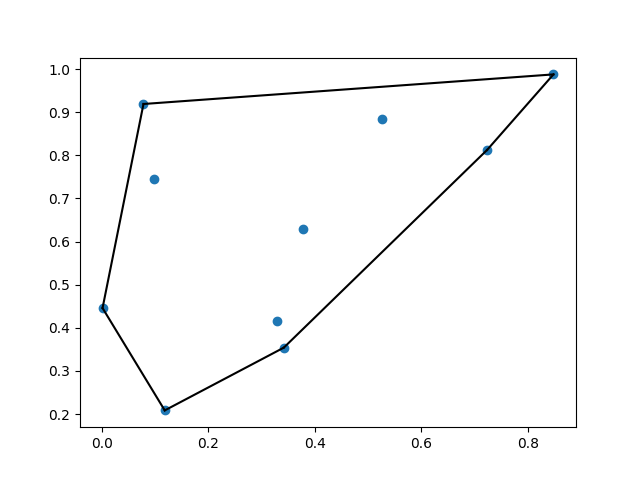`

`Lambda Runtime API`

`You can now select a custom runtime when creating or updating a function:`

`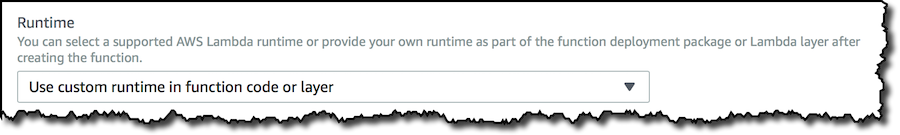`

`With this selection, the function must include (in its code or in a layer) an executable file called bootstrap, responsible for the communication between your code (that can use any programming language) and the Lambda environment.`

`The runtime bootstrap uses a simple HTTP based interface to get the event payload for a new invocation and return back the response from the function. Information on the interface endpoint and the function handler are shared as environment variables.`

`For the execution of your code, you can use anything that can run in the Lambda execution environment. For example, you can bring an interpreter for the programming language of your choice.`

`You only need to know how the Runtime API works if you want to manage or publish your own runtimes. As a developer, you can quickly use runtimes that are shared with you as layers.`

`We are making these open source runtimes available today:`

• `C++`
• `Rust`

`We are also working with our partners to provide more open source runtimes:`

• `Erlang (Alert Logic)`
• `Elixir (Alert Logic)`
• `Cobol (Blu Age)`
• `Node.js (NodeSource N|Solid)`
• `PHP (Stackery)`

`The Runtime API is the future of how we’ll support new languages in Lambda. For example, this is how we built support for the Ruby language.`

`Available Now`

`You can use runtimes and layers in all regions where Lambda is available, via the console or the AWS Command Line Interface (CLI). You can also use the AWS Serverless Application Model (SAM) and the SAM CLI to test, deploy and manage serverless applications using these new features.`

`There is no additional cost for using runtimes and layers. The storage of your layers takes part in the AWS Lambda Function storage per region limit.`

`To learn more about using the Runtime API and Lambda Layers, don’t miss our webinar on December 11, hosted by Principal Developer Advocate Chris Munns.`

`I am so excited by these new features, please let me know what are you going to build next!`